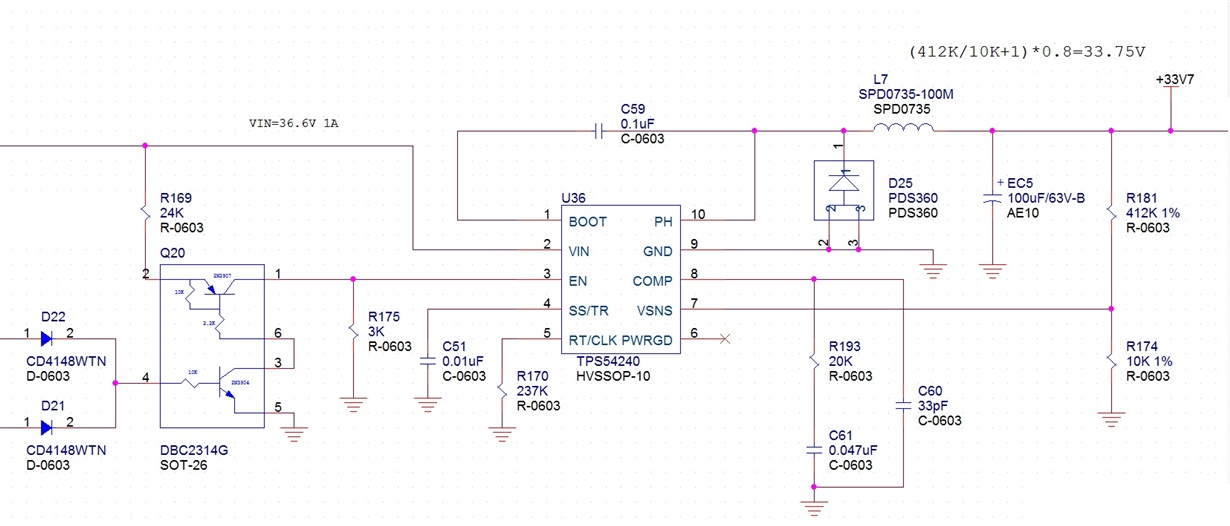If you have a related question, please click the "Ask a related question" button in the top right corner. The newly created question will be automatically linked to this question.

# TPS54240: How to set the two external resistors for EN pin

Part Number: TPS54240
Other Parts Discussed in Thread: TEST

about equation 2 & 3 of page 15 of TPS54240 datasheet

R1=(Vstart-Vstop)/Ihys

R2=Vena/((Vstart-Vena)/R1+I1)

Q1:

What is Vstart, Vstop and Vena?

Q2:

If I want to design a power application about 37V~40V input and 34V 1A output. How to set the two external resistors for EN pin?

Peter

• Hi Peter,
Equations that you are asking about are provided to calculate values if modified UVLO voltage is desired.
Vstart: input voltage needed to regulate output to 1% of set value
Vstop: input voltage where outputs drops 5% from regulation set point or device stops switching

For your particular case described above many resistor combinations can be used.
The main thing to keep in mind is that minimum required voltage on EN pin should be >1.15V and maximum should be <5V.
One of the resistor combinations for your Vin requirements could be Rtop=105k/Rbot=4.4k for Ven~1.5V for minimum input voltage.

I hope this helps.
Regards
Brani
• Vstart and Vstop is the desired threshold of Vin when you want the part to start operating (Vstart) and stop operating (Vstop)

Vena is the enable threshold voltage, which you can find from the electrical characteristic table.

To set your EN pin resistor, you should select you desired turn on turn off Vin voltage first.

-Yang

• I set Rtop=105k/Rbot=4.4k, but the TPS54240 is turn off, then I set Rtop=24k/Rbot=3k. the TPS54240 is turn on and EN=1.9v. Why? The drawings are as follows‧• Hi

For your application,the Vin is 37~40V. So you can set the Vstart=36V,   Vstop=35V. It means that when the Vin more than 36V, the IC turns on, when the Vin below 35V, the IC turns off.

So the

R1=(Vstart-Vstop)/Ihys=1/2.9uA=344K

R2=Vena/((Vstart-Vena)/R1+I1)=1.25/((36-1.25)/344K+0.9uA)=12.26K

By the way , the typical of Vena is 1.25V, and min=1.15V ,max=1.36V, so you need to know the Vena's value of your IC if you want the Vin-turn on and the  Vin-turn on more accurate.

Thank you!

• I try R1=300k, R2=15k, but EN pin is only 0.7v. Why?

Is it related to Q20?

• What's the Vin? And you can test the resistor between pin  1 to pin 2 when the pin 4 in high level(the PNP is opened).

• Vin=36v

Q20 is enable(pin 4 in high)

pin 2 = pin 1 = EN = 0.7v

Then  I set Rtop=24k/Rbot=3k. the TPS54240 is turn on and EN=1.9v. Why?

• Disconnect the divider resistor and EN pin, then power on to see what's the voltage of the pin 1 of Q20.

• I think that i find the solution of  problem. the divider resistor must include the inside resistor of Q20. Thank you very much.

• Yes, That's why I want you to test the resistor of the Q20.